# GSEB Solutions Class 12 Maths Chapter 6 Application of Derivatives Miscellaneous Exercise

Gujarat Board GSEB Textbook Solutions Class 12 Maths Chapter 6 Application of Derivatives Miscellaneous Exercise Textbook Questions and Answers.

## Gujarat Board Textbook Solutions Class 12 Maths Chapter 6 Application of Derivatives Miscellaneous ExerciseQuestion 1.
(a) ($$\frac { 17 }{ 81 }$$)$$\frac { 1 }{ 2 }$$
Solution: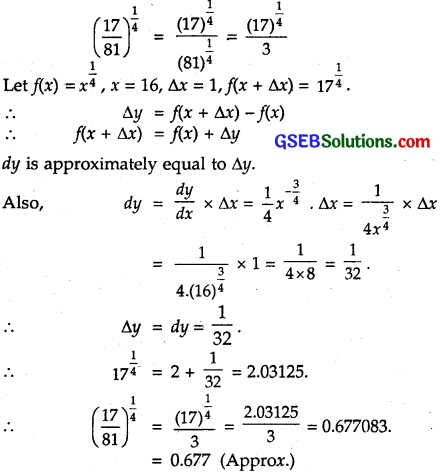(b) (33)$$\frac { 1 }{ 5 }$$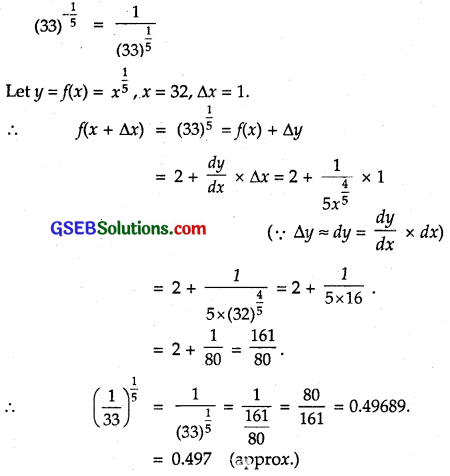:

Question 2.
Show that the functions given by f(x) = $$\frac{\log x}{x}$$ has a maximum at x = e.
Solution: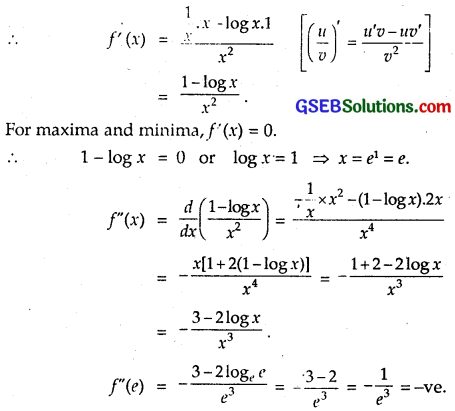∴ f is maximum at x = e.

Question 3.
The two equal sides of an isosceles triangle with fixed base b are decreasing at the rate of 3 cm per second. How fast is the area decreasing, when the two equal sides are equal to the base?
Solution:
Let x be the equal sides A of isosceles triangle with fixed base b, i.e., AB = AC = x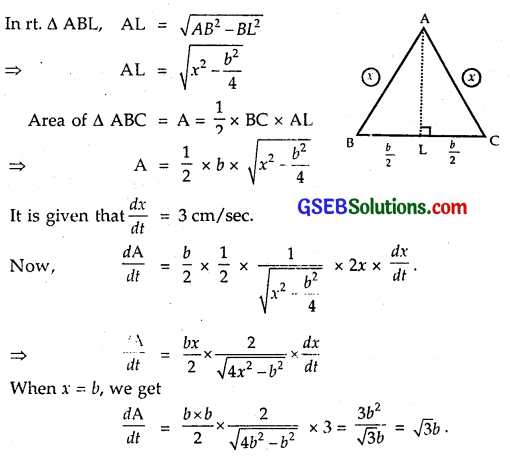Hence, the area is decreasing at $$\sqrt{3b}$$ cm²/sec.

Question 4.
Find the equation of the normal to the curve y² = 4x at the point (1, 2).
Solution:
The curve ìs y² = 4x.
Differentiating w.r.t. x, we get
2y $$\frac { dy }{ dx }$$ =4 or $$\frac { dy }{ dx }$$ = $$\frac { 4 }{ 2y }$$ = $$\frac { 2 }{ y }$$.
At(1, 2), $$\frac { dy }{ dx }$$ = $$\frac { 2 }{ 2 }$$ = 1.
∴ Slope of normal = – 1.
Equation of normal at (1, 2) is
y – 2 =(- 1)(x – 1)
or y – 2 = – x + 1 or x + y – 3 = 0.

Question 5.
Show that the normal at any point θ to the curve x = a cos θ + aθ sin θ, y = a sin θ – aθ cos θ is at a constant distance from the origin.
Solution:
We have: y = a cos θ + a θ sin θ
and x = a sin θ – a θ cos θ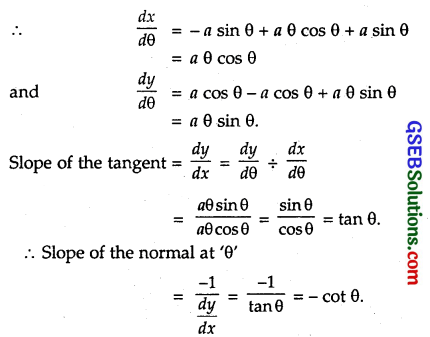∴ The equation of the normal at the point ‘θ’ is
[y – (a sin θ – a θ cos θ)] = – cot θ. [x – (a cos θ + a θ sin θ)].
On simplifying, we get x cos θ + y sin θ = a, which is the equation of the normal.
The distance of this normal from the origin
= $$\frac{a}{\sqrt{\cos ^{2} \theta+\sin ^{2} \theta}}$$
= a = constant.

Question 6.
Find the intervals in which the function f given by f(x) = $$\frac{4 \sin x-2 x-x \cos x}{2+\cos x}$$ is (i) increasing, (ii) decreasing.
Solution:
We have: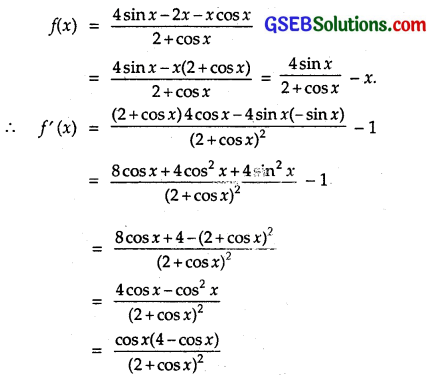Since – 1 ≤ cos x ≤ 1, therefore,
4 – cos x > 0 and also (2 + cos x)² > 0.
∴ f(x) > 0 or < 0 according as cos x > 0 or cos x < 0 respectively.
∴ f(x) is (i) increasing when 0 < x < $$\frac { π }{ 2 }$$, $$\frac { 3π }{ 2 }$$ < x < 2π and (ii) decreasing when $$\frac { π }{ 2 }$$ < x < $$\frac { 3π }{ 2 }$$.

Question 7.
Find the interval in which the function f given by f(x) = x³ + $$\frac{1}{x^{3}}$$, x ≠ 0 is (i) increasing, (ii) decreasing.
Solution:
We have : f(x) = x³ + $$\frac{1}{x^{3}}$$, x ≠ 0
∴ f'(x) = 3x² – $$\frac{3}{x^{4}}$$
For f(x) to be an increasing function of x,Hence, f(x) is increasing when x < – 1 and x > 1.
For f(x) to be decreasing function of x,
f'(x) < 0, i.e., 3(x² – $$\frac{1}{x^{4}}$$) < 0.
⇒ x² – $$\frac{1}{x^{4}}$$ < 0
⇒ x6 – 1 < 0.
⇒ (x³ – 1)(x³ + 1) < 0, which is possible when, (a) x³ – 1 > 0 and x³ + 1 < 0.
or (b) x³ – 1 < 0 and x³ + 1 > 0.

(a) x³ > 1 and x³ < – 1 ⇒ x > 1 and x < -1 not admissible.
(b) x³ < 1 and x³ > – 1 ⇒ x < 1 and x > – 1, i.e., – 1 < x < 1.
Hence, f(x) is decreasing when – 1 < x < 1.

Question 8.
Find the maximum area of an isosceles triangle inscribed x² y² in the ellipse $$\frac{x^{2}}{a^{2}}+\frac{y^{2}}{b^{2}}$$ = 1 with its vertex at one end of the major axis.
Solution:
A = area of isosceles ∆ APP’
= $$\frac { 1 }{ 2 }$$ . PP’. AM(where P and P’ are points on ellipse and A is a point where the ellipse intersect the major axis.)
= $$\frac { 1 }{ 2 }$$ab(2bsin θ )(a – a cos θ )
= ab(sin θ – $$\frac { 1 }{ 2 }$$sin 2θ)
∴ $$\frac { dA }{ dθ }$$ = ab(cos θ – cos 2θ)
For maxima and minima,
$$\frac { dA }{ dθ }$$ = 0.
∴ ab(cos θ – cos 2θ) = 0.
⇒ cos 2θ = cos θ.
⇒ θ = 2π – θ
⇒ θ = $$\frac { 2π }{ 3 }$$.Question 9.
A tank with rectangular base and rectangular sides, open at the top, is to be constructed so that its depth is 2 m and volume is 8 m3. If building of tank costs ₹ 70 per sq. metre for the base and ₹ 45 per m² for sides, what is the cost of least expensive tank?
Solution:
Let the length and breadth of the tank be x metres and y metres respectively. The depth of it is 2 metres.∴ Volume of tank = 2 × x × y = 2xy = 8
∴ xy = 4
Now, area of base = xy
and area of sides = 2.2(x + y) = 4 (x + y).
∴ Cost of construction C = ₹ [70 xy + 45 x 4(x + y)]
= ₹ [70 xy + 180(x + y) … (2)
Putting value of y, i.e., y = $$\frac { 4 }{ x }$$ in (2) from (1), we getFor maximum or minimum. $$\frac { dC }{ dx }$$ = 0.
⇒ x² – 4 = 0 ⇒ x = ± 2.
$$\frac { dC }{ dx }$$ changes sign from – ve to + ve at x = 2.
∴ C is minimum at x = 2. [Length of the tank cannot be negative)
∴ x = 2 and y = $$\frac { 4 }{ x }$$ = $$\frac { 4 }{ 2 }$$ = 2.
∴ Thus, tank is a cube of side 2 m.
∴ Least cost of construction
= ₹ [280 + 190(2 + $$\frac { 4 }{ 2 }$$)]
= ₹ 280 + 720 = ₹ 1000.

Question 10.
The sum of perimeters of a circle and a square is k, where k is some constant. Prove that the sum of their areas is least, when the side of square is double the radius of the circle.
Solution:
Let x be the radius of the circle and y be the side of the square.
Circumference of the circle = 2πx
and perimeter of square = 4y.
Sum of perimeters of circle and square = 2πx + 4y = k … (1)
Area of circle = π²
and area of square = y²
Sum of areas of circle and square = πx² + y²Question 11.
A window is in the form of a rectangular opening surmounted by a semi-circular opening. The total perimeter of the, window is 10 m. Find the dimensions of the window to admit maximum light through the whole opening.
Solution:
Perimeter of the window, when the width of window is x and 2r is the length
= 2x + 2r + $$\frac { 1 }{ 2 }$$ x 2πr = 10 [Given]
⇒ 10 = (π + 2)r + 2x … (1)
For admitting the maximum light through the whole opening, the area of the window must be maximum.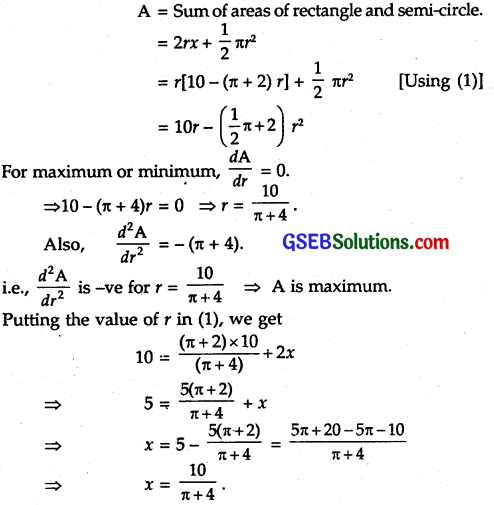∴ length of rectangle = 2r = $$\frac { 20 }{ π +4 }$$ and breadth = $$\frac { 10 }{ π+4 }$$

Question 12.
A point on the hypotenuse of a right triangle is at distance a and b from the sides of the triangle. Show that the minimum length of the hypotenuse is $$\left(a^{\frac{2}{3}}+b^{\frac{2}{3}}\right)^{\frac{3}{2}}$$
Solution:
Let P be a point on the hypotenuse AC of rt. ∠d ∆ABC such that PL (⊥AB) = a and PM(⊥ BC) = b.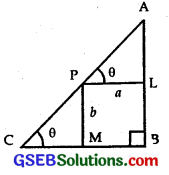Let ∠APL = ∠ACB = θ (say).
∴ AP = a(sec θ . sec θ + tan θ . sec θ tan )
Let l be the length of the hypotenuse. Then,Since, 0 < θ < $$\frac { π }{ 2 }$$, so all t-ratios of θ are positive. Also, a > 0 and b > 0.
∴ $$\frac{d^{2} l}{d \theta^{2}}$$ is positive.
⇒ l is least when tan θ = ($$\frac { b }{ a }$$)$$\frac { 1 }{ 3 }$$
∴ Least value of l = a sec θ + b cosec θ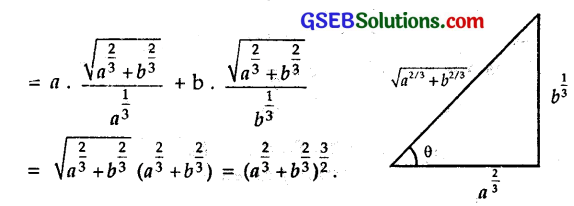Question 13.
Find the points at which the function f given by f(x) = (x – 2)4(x + 1)³ has (i) local maxima, (ii) local minima, (iii) point of inflection.
Solution:
Let
f(x) = (x – 2)4(x + 1)³
f ‘(x) = (x-2)4.3(x + 1)² + (x + 1)³.4(x – 2)³
= (x – 2)³ (x + 1)²[3(x – 2) + 4(x + 1)]
= (x-2)³(x+ 1)²(7x – 2)
= 7(x-2)³(x + 1)²
For maxima and minima, f'(x) = 0.
⇒ 7(x – 2)³(x + 1)²(x – $$\frac { 2 }{ 7 }$$) = 0.
⇒ x = 2, – 1, $$\frac { 2 }{ 7 }$$
At x = 2:
When x is slightly < 2, f’(x) = (-)(+)(+) =-ve. When x is slightly > 2, f'(x) = (+)(+)(+) = +ve.
⇒ f ‘(x) changes its sign from -ve to +ve while crossing the point x = 2.
⇒ f(x) is minimum at x = 2.

At x = – 1:
When x is slightly < – 1, f'(x) = (-)(+)(-) = +ve. When x is slightly > – 1, f ix) = (-)(+)(-) = +ve,
⇒ f'(x) does not change its sign, while passing through – 1.
Thus, x = – 1 is a point of infletion.

At x = $$\frac { 2 }{ 7 }$$:
When x is slightly < $$\frac { 2 }{ 7 }$$, f'(x) = (-)(+)(-) = +ve. When x is slightly > $$\frac { 2 }{ 7 }$$, f(x) = (-)(+)(+) = -ve.
⇒ f(x) changes its sign from +ve to -ve while crossing the point x = $$\frac { 2 }{ 7 }$$
∴ f(x) is maximum at x = $$\frac { 2 }{ 7 }$$.

Question 14.
Find the absolute maximum and minimum values of the function f given by f(x) = cos² x + sin x, x ∈ [0, π].
Solution:
Let f(x) = cos² x + sin x, x ∈ (0, π).
∴ f(x) = 2 cos x (- sin x) + cos x .
= cos x (- 2 sin x + 1).
For maximum or minimum, f'(x) = 0.
∴ cos x(- 2 sin x + 1) = 0.Of these values, the maximum and minimum values of f(x) are $$\frac { 5 }{ 4 }$$ and 1 respectively.
So, absolute maximum = $$\frac { 5 }{ 4 }$$ and absolute minimum = 1.

Question 15.
Show that the altitude of the right circular cone of maximum volume that can be inscribed in a sphere of radius r is $$\frac { 4r }{ 3 }$$.
Solution:
The radius of sphere is r in which a cone is inscribed. Let O be the centre of the sphere. BC is the diameter of the base of cone. Let AM = Altitude, ∠ BOM = θ. Therefore, radius of the cone R = r sin θ.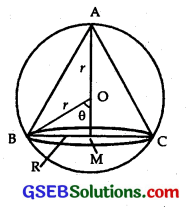Altitude of the cone ABC = AM = AO + OM = r + r cos θ = r(l + cos θ)
Volume of the cone V = $$\frac { 1 }{ 3 }$$ πR²H = $$\frac { 1 }{ 3 }$$ πr²sin² θ x r(1 + cos θ)$$\frac { dV }{ dθ }$$ changes sign from +ve to -ve [+ + + = +ve, ++ – = -ve], while crossing through the point θ = cos-1$$\frac { 1 }{ 3 }$$ .
∴ V is maximum at cos θ = $$\frac { 1 }{ 3 }$$.
So, altitude = r(1 + cos θ) = r(1 + $$\frac { 1 }{ 3 }$$) = $$\frac { 4r }{ 3 }$$.

Question 16.
Let f be a function defined on [a, b} such that f’ (x) > 0 for all x ∈ (a, b). Then, prove that/is an increasing function on (a, b).
Solution:
Let x1, x2 ∈ (a, b) such that x1 <x2
Consider the sub-interval [x1, x2].
Since f(x) is differentiable on (a, b) and [x1, x2] ⊂ (a, b), therefore
f(x) is continuous on [x1, x2] and differentiable on (x1, x2).
By the Langrange’s mean value theorem, there exists c ∈ (x1, x2) such that f'(c) = $$\frac{f\left(x_{2}\right)-f\left(x_{1}\right)}{x_{2}-x_{1}}$$. Since f'(x) > 0 for all x ∈ (a, b), so in particular, f'(c) > 0.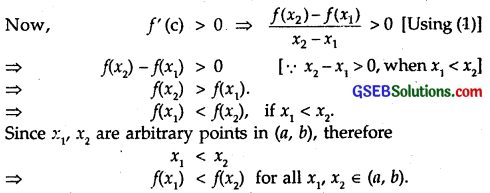Hence, f(x) is increasing on (a, b).

Question 17.
Show that the height of the cylinder of maximum volume that can be inscribed in a sphere of radius R is $$\frac{2 R}{\sqrt{3}}$$. Also,, find the maximum volume.
Solution:
Radius of the sphere = R.
Let h be the height and x be the diameter of the base of the inscribed cylinder. Then,
h² + x² = (2R)²
⇒ h² + x² = 4R² … (1)
Volume of the cylinder = π(radius)² x height.Question 18.
Show that height of the cylinder of greatest volume which can be inscribed in a right circular cone of height ft and having semi-vertical angle a is one-third that of the cone and the greatest volume of cylinder is $$\frac { 4 }{ 27 }$$ πh³ tan² α.
Solution:
Let VAB be the cone of height h, semi-vertical angle a and let x be the radius of the base of the cylinder A’B’DC which is inscribed in the cone VAB. Then,Height (OO’) of the cylinder = VO – VO’ = h – x cot α
Volume V of the cylinder = πr² (h – x cot α) …(1)
∴ $$\frac { dV }{ dx }$$ = 2πxh – 3πx² cot α.
For maxima or minima of V, $$\frac { dV }{ dx }$$ = 0.
⇒ 2πxh – 3πx²cotα = 0.
⇒ x = $$\frac { 2h }{ 3 }$$ tan α. [∵ x ≠ 0]
Now, $$\frac{d^{2} V}{d x^{2}}$$ = 2πh – 6πr cot α.
When x = $$\frac { 2h }{ 3 }$$ tan α, we have :Question 19.
A cylindrical tank of radius 10 feet is being filled with wheat at the rate of 314 cubic feet per minute. Then, the depth of the wheat is increasing at the rate of
(A) 1 cubic ft/minute
(B) 0.1 cubic ft/minute
(C) 1.1 cubic feet/minute
(D) 0.5 cubic ft/minute
Solution:
Let h be the height (depth) of the cylindrical tank at any instant.
Volume of the cylinderical tank = πr²h [∵ r = 10 ft]
∴ V = 100 Th.
Rate of change of volume = $$\frac { dV }{ dt }$$
Now, $$\frac { dV }{ dt }$$ = 100 π $$\frac { dh }{ dt }$$ … (1)
i.e. $$\frac { dV }{ dt }$$ = 314.
From (1), 314 = 100 π $$\frac { dh }{ dt }$$
∴ $$\frac { dh }{ dt }$$ = $$\frac { 314 }{ 100π }$$ = $$\frac { 314 }{ 100×3.14 }$$ = 1.
Hence, the depth of the tank changes at 1 cubic ft/minute.
∴ Part (A) is the correct answer.

Question 20.
The slope of the tangent to the curve x = t² + 3t – 8, y = 2t² – 2t – 5 at the point (2, -1) is
(A) $$\frac { 22 }{ 7 }$$
(B) $$\frac { 6 }{ 7 }$$
(C) $$\frac { 7 }{ 6 }$$
(D) $$\frac { -6 }{ 7 }$$
Solution:
The curve is x = t² + 3t – 8, y = 2t² – 2t – 5 … (1)
Putting x = 2, 2 = t² + 3t – 8 ∴ t² + 3t – 10 = 0
or (t + 5)(t – 2) =0
Putting t = 2 in y = 2t² – 2t – 5 = 8 – 4 – 5 = – 1
∴ At x = 2, y = – 1 and t = 2.
Differentiating (1) w.r.t. to t, we get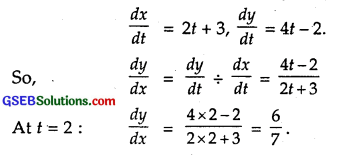∴ Part (B) is the correct answer.

Question 21.
The line y = mx + 1 is a tangent to the curve y² = 4x, if the value of m is
(A) 1
(B) 2
(C) 3
(D) $$\frac { 1 }{ 2 }$$
Solution:
The equation of the curve is y² = 4x.
Differentiating w.r.t. x, we get
2y$$\frac { dy }{ dx }$$ = 4
∴ $$\frac { dy }{ dx }$$ = $$\frac { 4 }{ 2y }$$ = $$\frac { 2 }{ y }$$.
∴ Slope of the tangent = $$\frac { 2 }{ y }$$ = m
∴ y = $$\frac { 2 }{ m }$$ … (1)
(x1, y1) lies on y² = 4x.
∴ y²1 = 4x1 … (2)
∴ Equation of tangent at (x1, y1) is
y – y1 = m(x – x1) = mx – mx1 … (3)
or y = mx + y1 – mx1
We are given the equation of tangent as y = mx + 1 … (4)
Comparing (3) and (4),
y1 – mx1 = 1 … (5)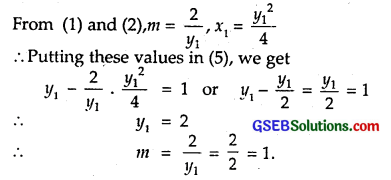∴ Part (A) is the correct answer.

Question 22.
The normal at the point (1,1) on the curve 2y + x² = 3 is
(A) x + y = 0
(B) (x – y) = 0
(C) x + y +1 = 0
(D) x – y = 0
Solution:
The equation of the curve is 2y + x² = 3.
Differentiating w.r.t. x, we get
2$$\frac { dy }{ dx }$$ + 2x = 0 ⇒ $$\frac { dy }{ dx }$$ = – x.
$$\frac { dy }{ dx }$$ at (1, 1) = – 1 = slope of tangent.
Therefore, slope of normal = $$\frac{1}{\text { slope of tangent }}$$ = 1.
∴ The equation of the normal is
y – y1 = (slope of normal)(x – x1)
y – 1 = 1 . (x – 1) or y – 1 = x – 1
or x – y = 0.
∴ Part (B) is the correct answer.

Question 23.
The normal to the curve x² = 4y passing through (1, 2) is
(A) x + y = 3
(B) x – y = 3
(C) x + y = 1
(D) x – y = 1
Solution:
Equation of the curve is x² = 4y.
Differentiating, w.r.t. x, we get
2x = 4$$\frac { dy }{ dx }$$.
∴ $$\frac { dy }{ dx }$$ = $$\frac { 2x }{ 4 }$$ = $$\frac { x }{ 2 }$$ = slope of the tangent.Putting these values in (1), equation of normal is
y – 1 = $$\frac { -2 }{ 2 }$$(x – 2)
or y – 1 = – x + 2 or x + y = 3.
∴ Part (A) is the correct answer.

Question 24.
The points on the curve 9y² = x³, where the normal to the curve make equal intercepts with axes are
(A) $$\left(4, \pm \frac{8}{3}\right)$$
(B) $$\left(\pm 4,-\frac{8}{3}\right)$$
(C) $$\left(4, \pm \frac{3}{8}\right)$$
(D) $$\left(\pm 4, \frac{3}{8}\right)$$
Solution:
The equation of the curve is 9y² = x³.
Differentiating w.r.t. x, we get
18y$$\frac { dy }{ dx }$$ = 3x² ⇒ $$\frac { dy }{ dx }$$ = $$\frac{x^{2}}{6 y}$$.
Let P(x1, y1) be the point, where normal is drawn.
Slope of tangent = $$\frac{x_{1}^{2}}{6 y_{1}}$$.
Slope of normal = – $$\frac{x_{1}^{2}}{6 y_{1}}$$.
Normal make equal intercepts on the curve.
∴ Its slope = ± 1.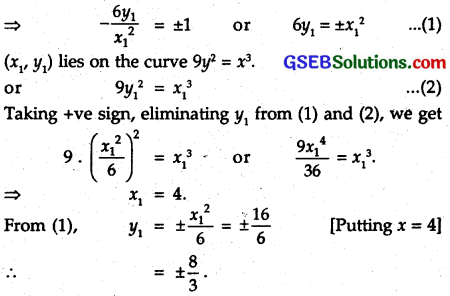∴ The points P are (4, ± $$\frac { 8 }{ 3 }$$).
∴ Part (A) is the correct answer.